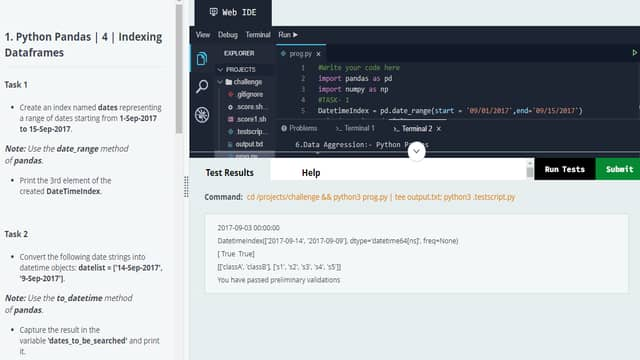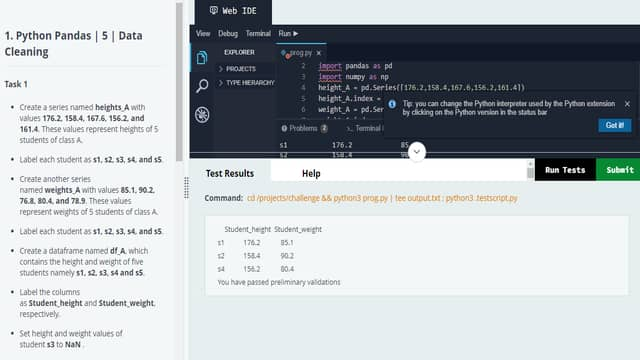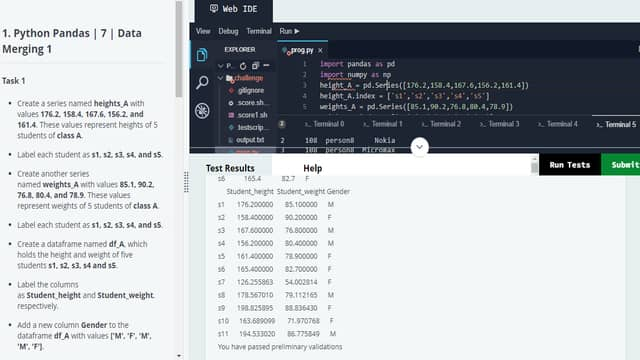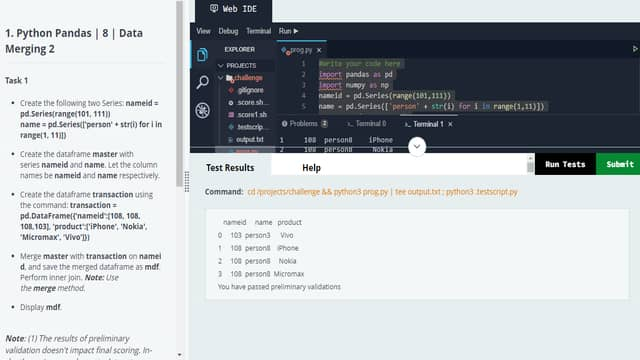# Python Pandas Hands-on Solutions | TCS Fresco Play

1Python Pandas Fresco Play Hands-on Solutions

Disclaimer: The primary purpose of providing this solution is to assist and support anyone who are unable to complete these courses due to a technical issue or a lack of expertise. This website's information or data are solely for the purpose of knowledge and education.

Make an effort to understand these solutions and apply them to your Hands-On difficulties. (It is not advisable that copy and paste these solutions).

Course Path: Data Science/DATA SCIENTIST'S TOOLBOX/Python Pandas

All Question of the Quiz Present Below for Ease Use Ctrl + F to find the Question.

Suggestion: If you didn't find the question, Search by options to get a more accurate result.

1.Welcome to Python Pandas | 1 | Data Structures in Pandas(60 Min)

Pandas Data Structures - Hands-onFile Name: prog.py

import pandas as pd

import numpy as np

heights_A = pd.Series([176.2,158.4,167.6,156.2,161.4])

heights_A.index = ['s1','s2','s3','s4','s5']

print(heights_A.shape)

weights_A = pd.Series([85.1,90.2,76.8,80.4,78.9])

weights_A.index = ['s1','s2','s3','s4','s5']

print(weights_A.dtype)

df_A = pd.DataFrame()

df_A['Student_height'] = heights_A

df_A['Student_weight'] = weights_A

print(df_A.shape)

my_mean = 170.0

my_std = 25.0

np.random.seed(100)

heights_B = pd.Series(np.random.normal(loc = my_mean, scale = my_std, size = 5))

heights_B.index = ['s1','s2','s3','s4','s5']

my_mean1 = 75.0

my_std1 = 12.0

weights_B = pd.Series(np.random.normal(loc = my_mean1,scale = my_std1,size = 5))

weights_B.index = ['s1','s2','s3','s4','s5']

print(heights_B.mean())

df_B = pd.DataFrame()

df_B['Student_height'] = heights_B

df_B['Student_weight'] = weights_B

print(df_B.columns)

2.Welcome to Python Pandas | 3 | Working with CSV files(90 min)

Working with CSVsFile Name: prog.py

#Write your code here

import pandas as pd

import numpy as np

heights_A = pd.Series([176.2,158.4,167.6,156.2,161.4])

heights_A.index = ['s1','s2','s3','s4','s5']

weights_A = pd.Series([85.1,90.2,76.8,80.4,78.9])

weights_A.index = ['s1','s2','s3','s4','s5']

df_A = pd.DataFrame()

df_A['Student_height'] = heights_A

df_A['Student_weight'] = weights_A

df_A.to_csv('classA.csv')

print(df_A2)

df_A3 = pd.read_csv('classA.csv',index_col = 0)

print(df_A3)

my_mean = 170.0

my_std = 25.0

np.random.seed(100)

heights_B = pd.Series(np.random.normal(loc = my_mean, scale = my_std, size = 5))

heights_B.index = ['s1','s2','s3','s4','s5']

my_mean1 = 75.0

my_std1 = 12.0

np.random.seed(100)

weights_B = pd.Series(np.random.normal(loc = my_mean1,scale = my_std1,size = 5))

weights_B.index = ['s1','s2','s3','s4','s5']

df_B = pd.DataFrame()

df_B['Student_height'] = heights_B

df_B['Student_weight'] = weights_B

df_B.to_csv('classB.csv',index = False)

print('classB.csv')

print(df_B2)

print(df_B3)

df_B4 = pd.read_csv('classB.csv',header = None, skiprows = 2)

print(df_B4)

3.Welcome to Python Pandas | 4 | Indexing Dataframes(75 Min)

Hands-on with IndexesFile Name: prog.py

#Write your code here

import pandas as pd

import numpy as np

DatetimeIndex = pd.date_range(start = '09/01/2017',end='09/15/2017')

print(DatetimeIndex)

datelist = ['14-Sep-2017','09-Sep-2017']

date_to_be_searched = pd.to_datetime(datelist)

print(date_to_be_searched)

print(date_to_be_searched.isin(datelist))

arraylist = [['classA']*5 + ['classB']*5,['s1','s2','s3','s4','s5']* 2]

mi_index = pd.MultiIndex.from_product(arraylist,names=['First Level','Second Level'])

print(mi_index.levels)

4.Welcome to Python Pandas | 5 | Data Cleaning

Data Cleaning - HandsonFile Name: prog.py

#Write your code here

import pandas as pd

import numpy as np

height_A = pd.Series([176.2,158.4,167.6,156.2,161.4])

height_A.index = ['s1','s2','s3','s4','s5']

weight_A = pd.Series([85.1,90.2,76.8,80.4,78.9])

weight_A.index = ['s1','s2','s3','s4','s5']

df_A = pd.DataFrame()

df_A['Student_height'] = height_A

df_A['Student_weight'] = weight_A

df_A.loc['s3'] = np.nan

df_A.loc['s5'] = np.nan

df_A2 = df_A.dropna(how = 'any')

print(df_A2)

5.Welcome to Python Pandas | 6 | Data Aggregation(75 Min)

Data Aggregation - HandsonFile Name: prog.py

#Write your code here

import pandas as pd

import numpy as np

heights_A = pd.Series([176.2,158.4,167.6,156.2,161.4])

heights_A.index = ['s1','s2','s3','s4','s5']

weights_A = pd.Series([85.1,90.2,76.8,80.4,78.9])

weights_A.index = ['s1','s2','s3','s4','s5']

df_A = pd.DataFrame()

df_A['Student_height'] = heights_A

df_A['Student_weight'] = weights_A

df_A_filter1 = df_A[(df_A.Student_weight < 80.0) & (df_A.Student_height > 160.0)]

print(df_A_filter1)

df_A_filter2 = df_A[df_A.index.isin(['s5'])]

print(df_A_filter2)

df_A['Gender'] = ['M','F','M','M','F']

df_groups = df_A.groupby('Gender')

print(df_groups.mean())

6.Welcome to Python Pandas | 7 | Data Merging 1(75 Min)

Data Merge - Hands-on 1File Name: prog.py

import pandas as pd

import numpy as np

height_A = pd.Series([176.2,158.4,167.6,156.2,161.4])

height_A.index = ['s1','s2','s3','s4','s5']

weights_A = pd.Series([85.1,90.2,76.8,80.4,78.9])

weights_A.index = ['s1','s2','s3','s4','s5']

df_A = pd.DataFrame()

df_A['Student_height'] = height_A

df_A['Student_weight'] = weights_A

df_A['Gender'] = ['M','F','M','M','F']

s = pd.Series([165.4,82.7,'F'],index = ['Student_height','Student_weight','Gender'],name='s6')

df_AA = df_A.append(s)

print(df_AA)

my_mean = 170.0

my_std = 25.0

np.random.seed(100)

heights_B = pd.Series(np.random.normal(loc = my_mean,scale=my_std,size = 5))

heights_B.index = ['s1','s2','s3','s4','s5']

my_mean1 = 75.0

my_std1 = 12.0

np.random.seed(100)

weights_B = pd.Series(np.random.normal(loc = my_mean1,scale=my_std1,size = 5))

weights_B.index = ['s1','s2','s3','s4','s5']

df_B = pd.DataFrame()

df_B['Student_height'] = heights_B

df_B['Student_weight'] = weights_B

df_B.index=['s7','s8','s9','s10','s11']

df_B['Gender'] = ['F','M','F','F','M']

df = pd.concat([df_AA,df_B])

print(df)

7.Welcome to Python Pandas | 8 | Data Merging 2(75 Min)

Data Merge - Hands-on 2File Name: prog.py

#Write your code here

import pandas as pd

import numpy as np

nameid = pd.Series(range(101,111))

name = pd.Series(['person' + str(i) for i in range(1,11)])

master = pd.DataFrame()

master['nameid'] = nameid

master['name'] = name

transaction = pd.DataFrame({'nameid':[108,108,108,103],'product':['iPhone','Nokia','Micromax','Vivo']})

mdf = pd.merge(master,transaction,on='nameid')

print(mdf)

8.Welcome to Python Pandas | 2 | Accessing Pandas Data Structures(90 Min)

Access Elements in Data StructuresFile Name: prog.py

#Write your code here

import pandas as pd

import numpy as np

heights_A = pd.Series([176.2,158.4,167.6,156.2,161.4])

heights_A.index = ['s1','s2','s3','s4','s5']

print(heights_A)

print(heights_A[1:4])

weights_A = pd.Series([85.1,90.2,76.8,80.4,78.9])

weights_A.index = ['s1','s2','s3','s4','s5']

df_A = pd.DataFrame()

df_A['Student_height'] = heights_A

df_A['Student_weight'] = weights_A

height = df_A['Student_height']

print(type(height))

df_s1s2 = df_A[df_A.index.isin(['s1','s2'])]

print(df_s1s2)

df_s2s5s1 = df_A[df_A.index.isin(['s1','s2','s5'])]

df_s2s5s1 = df_s2s5s1.reindex(['s2','s5','s1'])

print(df_s2s5s1)

df_s1s4 = df_A[df_A.index.isin(['s1','s4'])]

print(df_s1s4)

If you have any queries, please feel free to ask on the comment section.

If you want MCQs and Hands-On solutions for any courses, Please feel free to ask on the comment section too.

1.Python Pandas MCQ Solution --> https://www.mncanswers.site/2022/03/python-pandas-mcq-solutions-tcs-fresco.html